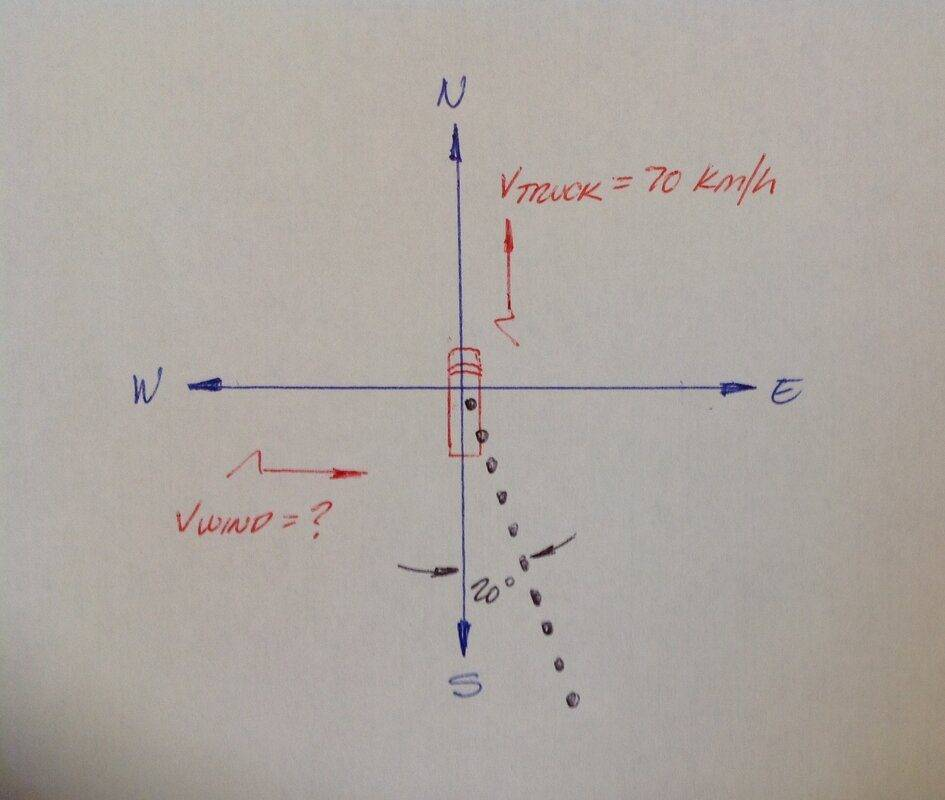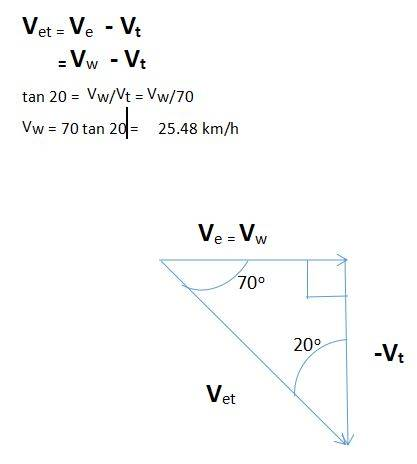# Does the exhaust of a truck match the velocity of the truck?

vcsharp2003
Homework Statement:
A truck is moving north at a speed of 70km/h. The exhaust pipe above the truck cab sends out a trail of smoke that makes an angle of 20 degrees east of south behind the truck. If the wind is blowing directly towards the east, what is the wind speed at that location?
Relevant Equations:
Ve + Vw = net velocity of exhaust, where Ve is velocity of exhaust as it comes out of the exhaust pipe and Vw is velocity of wind.
My doubt is whether its correct to assume that the exhaust is having the velocity of truck when it comes out of the exhaust pipe. So, I am not sure if Ve = Vt.

Should not the exhaust come out in a south direction from exhaust pipe since truck is moving in north direction? If yes, then it cannot have the velocity of truck when it comes out the exhaust pipe.

Homework Helper
Gold Member
For the purpose of this problem, you can consider any exhausted mass of combustion gas to remain at the spatial point at which it was exhausted, then, the wind starts pushing it Eastwards from that point.
If you could draw a horizontal line between that mass and the next one that came out from the moving North exhaust pipe, your line would form a 20° angle respect to the North-South line.

What angle would be formed if the velocity of the wind were 70 km/h West to East?

vcsharp2003
For the purpose of this problem, you can consider any exhausted mass of combustion gas to remain at the spatial point at which it was exhausted, then, the wind starts pushing it Eastwards from that point.
If you could draw a horizontal line between that mass and the next one that came out from the moving North exhaust pipe, your line would form a 20° angle respect to the North-South line.

What angle would be formed if the velocity of the wind were 70 km/h West to East?
If I use your assumption, then the velocity of exhaust when it comes out would be 70 km/h North relative to a ground observer. Then the wind velocity pushes this exhaust in an East direction. This would make the exhaust gas move with a velocity in some Northeast direction relative to ground observer, but the problem says the exhaust moves in some Southeast direction. Is my reasoning correct?

Also if wind blows from West to East at 70 km/h then exhaust will move in a Northwest direction at 45 degrees with North direction.

Homework Helper
Gold Member
If I use your assumption, then the velocity of exhaust when it comes out would be 70 km/h North relative to a ground observer. Then the wind velocity pushes this exhaust in an East direction. This would make the exhaust gas move with a velocity in some Northeast direction relative to ground observer, but the problem says the exhaust moves in some Southeast direction. Is my reasoning correct?

Also if wind blows from West to East at 70 km/h then exhaust will move in a Northwest direction at 45 degrees with North direction.
Only to simplify things, we are assuming that the velocity of the exhausted gases respect to the truck is 70 km/h Southward.
Even if that velocity of the exhaust were higher or lower, each puff of smoke would eventually slowdown and come to a still condition in the absence of that crosswind, again forming a line conditioned for the uniform time delays among consecutive spuffs of smoke.

Relative to a ground observer, the truck is moving North at 70 km/h and the velocity of its exhaust is initially zero; then he sees that puff of black smoke start being carried away Eastward by the wind.
Next puff will be exhausted a little further North and with some time delay, then behaving as previous one.

Just as a line is a set of points, the exhaust trail of our problem is a set of puffs of smoke.
The problem statement of "20 degrees east of south behind the truck" means that a line is foming an angle of 20° respect to the North-South line.

https://www.physicsclassroom.com/class/vectors/Lesson-1/Vectors-and-DirectionLast edited:
•vcsharp2003
vcsharp2003
Thanks for your reply. I think this is a simple problem of relative velocity. The exhaust velocity relative to truck is in a south east direction at 20 degrees to North South line. With your assumption, velocity of exhaust relative to ground is same as wind velocity. Then it becomes a simple problem of a right angled vector triangle.

•Lnewqban
Homework Helper
Gold Member
Yes, you have to pretend, rather unrealistically, that if the truck is not moving and the wind not blowing, the velocity of the exhaust gases is zero relative to the truck.

•jim mcnamara
Homework Helper
Gold Member
2022 Award
Yes, you have to pretend, rather unrealistically, that if the truck is not moving and the wind not blowing, the velocity of the exhaust gases is zero relative to the truck.
It says the exhaust pipe is above the truck. A vertical exhaust above the cabin is a common arrangement for trucks.

Homework Helper
Gold Member
It says the exhaust pipe is above the truck. A vertical exhaust above the cabin is a common arrangement for trucks.
Yes indeed but their ends are often angled so that the exhaust velocity has a horizontal component which itself is angled relative to the long axis of the truck.vcsharp2003
The problem is meaning that the velocity of smoke relative to truck is in a direction 20o east of south i.e. an observer in truck will see exhaust move in that direction. Then, Vet = Ve- Vt is vector equation for this problem and since exhaust will move with the wind so Ve = Vw. Therefore we can draw a vector triangle that is right angled using the vector equation Vet = Vw- Vt in which we know one angle of 20o and one side for Vt which is 70 km/h. We can then easily solve for Vw using simple trigonometry.

How one can conclude that velocity is relative to truck is as explained below.

An excellent diagram given by Lnewqban at https://www.physicsforums.com/threa...he-velocity-of-the-truck.1001112/post-6471415 shows how the trail of exhaust smoke is formed and how it moves. Each puff of exhaust is moving east at wind speed relative to tip of exhaust pipe and also at the same time moving south at 70 km/h relative to the tip of exhaust pipe. Then the explanation given in above paragraph can be used since we are sure now that we are measuring velocity relative to tip of exhaust pipe i.e. the truck.

Last edited:
•Lnewqban
Homework Helper
Gold Member
2022 Award
To me it seems that the problem is not precisely worded. The problem is meaning that the velocity of smoke relative to truck is in a direction 20o east of south
I see no problem with the wording. It says that if anyone were to take an overhead shot of the smoke trail at some instant they would see it as a line going 20° east of south from the truck. From that you can deduce that the smoke is moving that way relative to the truck.

•Lnewqban and vcsharp2003
vcsharp2003
I see no problem with the wording. It says that if anyone were to take an overhead shot of the smoke trail at some instant they would see it as a line going 20° east of south from the truck. From that you can deduce that the smoke is moving that way relative to the truck.
You are correct. I think its more appropriate to call this a difficult level problem since student is expected to analyze and then make correct conclusion of velocity being relative to truck. I have accordingly changed my post that mentioned this.

vcsharp2003
After using the great and very helpful posts in this thread, the following is the solution to this problem. We are looking at the velocity of a puff of exhaust relative to truck.

Thanks to all who helped me understand this problem.

I would label this as a difficult level problem since student has to analyze the problem and make the correct conclusions on how a puff of exhaust moves.

The answer is mentioned as 25 km/h for this problem.

SOLUTION•Lnewqban